Drop a Query

# Selva Prabhakaran

Selva is an experienced Data Scientist and leader, specializing in executing AI projects for large companies. Selva started machinelearningplus to make Data Science / ML / AI accessible to everyone. The website enjoys 4 Million+ readership. His courses, lessons, and videos are loved by hundreds of thousands of students and practitioners.## Scrapy vs. Beautiful Soup: Which is better for web scraping?

Web scraping is the technique of extracting data from a specific website or web page. This has wide applications in: Research and publication purposes Competitor and market studies Creating data for machine learning models The extracted data can be stored in any format be it a csv, txt, json, API etc so that it can …

## add Python to PATH – How to add Python to the PATH environment variable in Windows?

1. What is the purpose of adding Python to the PATH environment variable? Adding Python to the PATH environment variable in Windows allows you to run Python commands from any directory within the command prompt. Here are the steps to add Python to the PATH variable: 2. What is the PATH environment variable in Windows? …## MICE imputation – How to predict missing values using machine learning in Python

MICE Imputation, short for ‘Multiple Imputation by Chained Equation’ is an advanced missing data imputation technique that uses multiple iterations of Machine Learning model training to predict the missing values using known values from other features in the data as predictors. What is MICE Imputation? You can impute missing values by predicting them using other …## conda list environments – How to view all the virtual environments present in conda?

conda is a popular package management system that allows you to create isolated environments with different versions of packages and dependencies. In this one, let’s see how to view a list of all virtual environments in conda. How to list all the virtual Environments? Earlier you saw everything about creating conda environments. If you want …## conda delete environment – How to remove a conda environment and all the associated packages?

conda is a popular package management system that allows you to create isolated environments with different versions of packages and dependencies. Earlier we saw how to create a new conda environment and steps to manage it. However, if you no longer need an environment, you can delete it to free up disk space and simplify …## Spline Interpolation – How to find the polynomial curve to interpolate missing values

Spline interpolation is a special type of interpolation where a piecewise lower order polynomial called spline is fitted to the datapoints. That is, instead of fitting one higher order polynomial (as in polynomial interpolation), multiple lower order polynomials are fitted on smaller segments. This can be implemented in Python. You can do non-linear spline interpolation …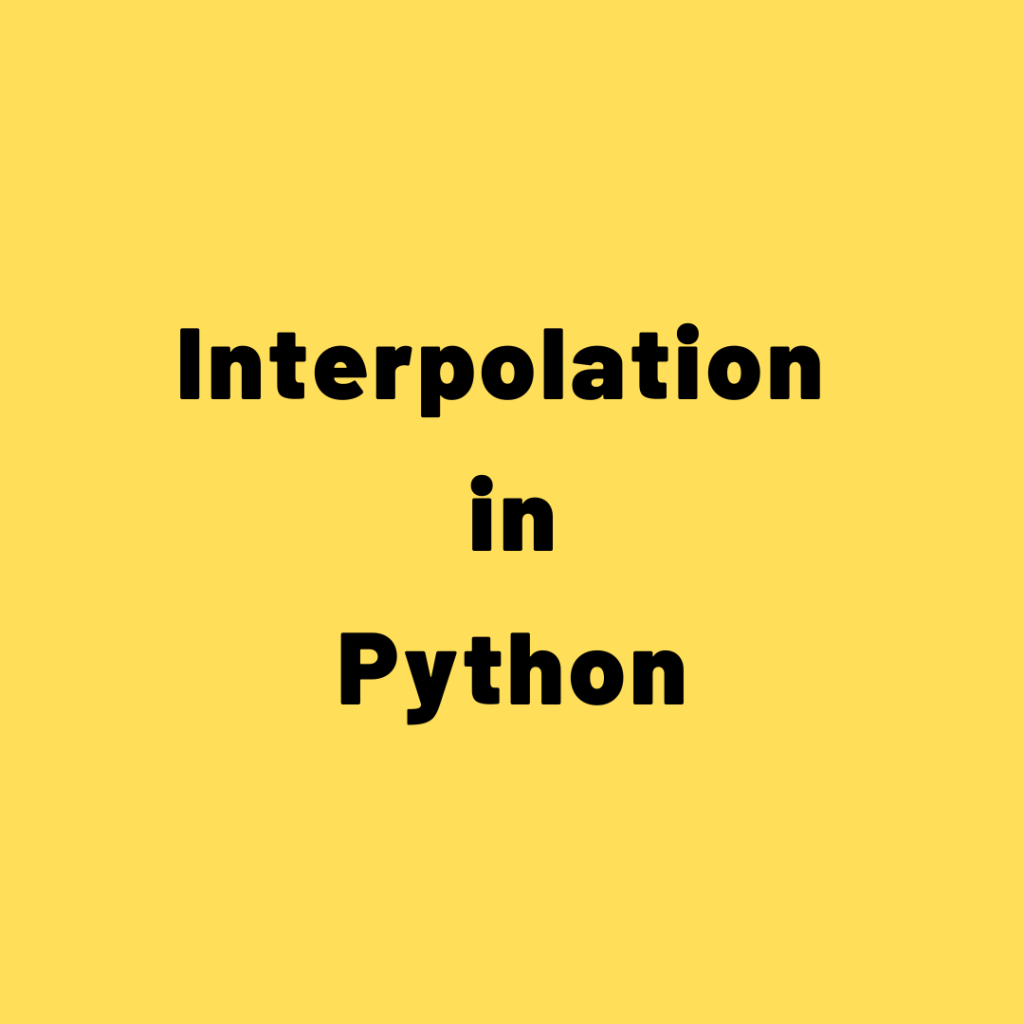## Interpolation in Python – How to interpolate missing data, formula and approaches

Interpolation can be used to impute missing data. Let’s see the formula and how to implement in Python. But, you need to be careful with this technique and try to really understand whether or not this is a valid choice for your data. Often, interpolation is applicable when the data is in a sequence or …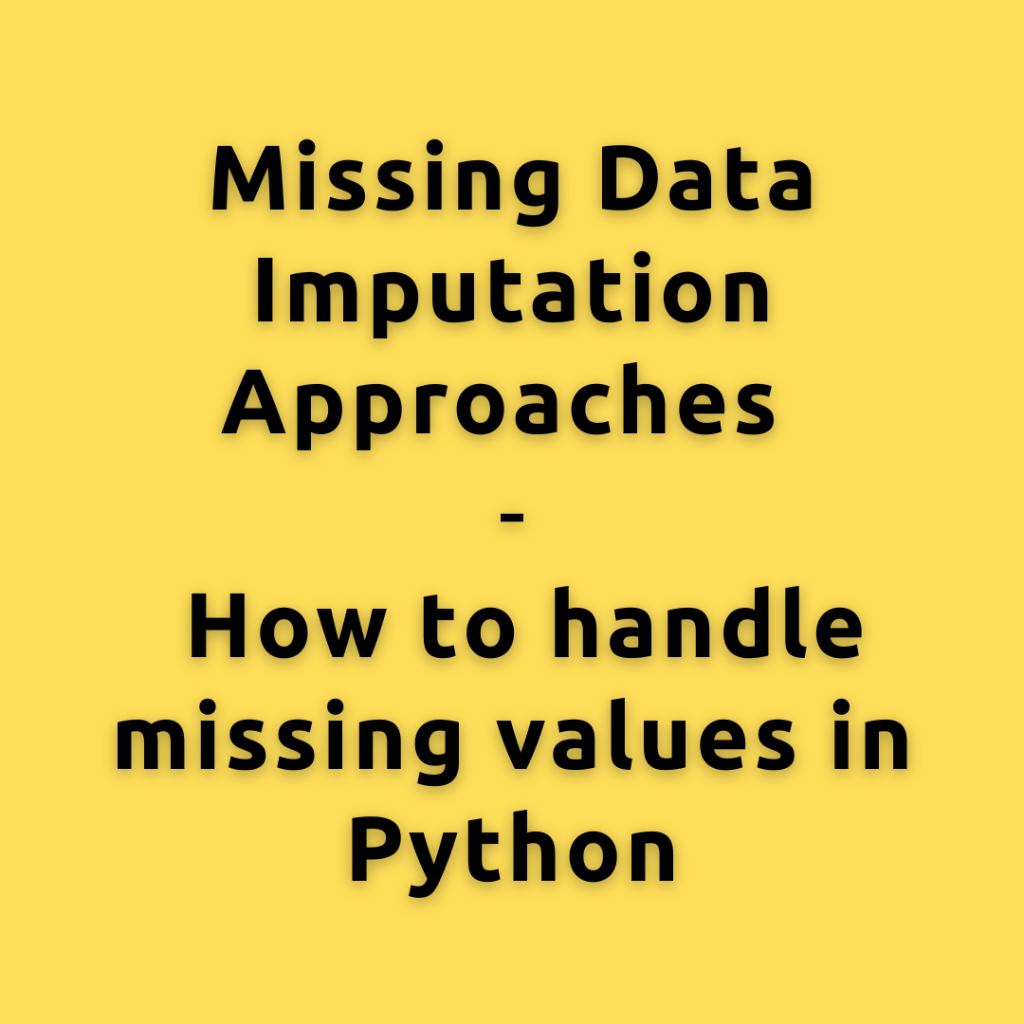## Missing Data Imputation Approaches | How to handle missing values in Python

Machine Learning works on the idea of garbage in – garbage out. If you put in useless junk data to the machine learning algorithm, the results will also be, well, ‘junk’. The quality and consistency of results depend on the data provided. Missing values in data degrade the quality. Why clean the data before training …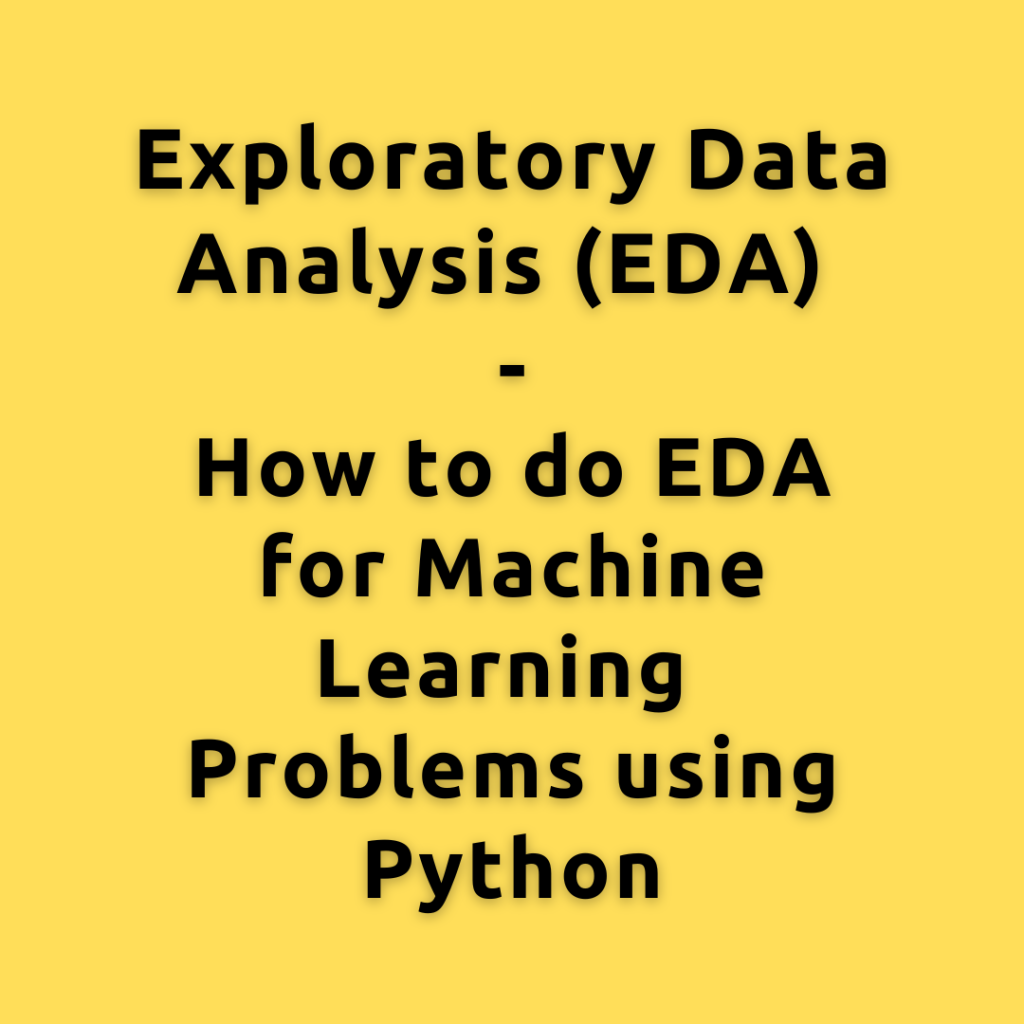## Exploratory Data Analysis (EDA) – How to do EDA for Machine Learning Problems using Python

Exploratory Data Analysis, simply referred to as EDA, is the step where you understand the data in detail. You understand each variable individually by calculating frequency counts, visualizing the distributions, etc. Also the relationships between the various combinations of the predictor and response variables by creating scatterplots, correlations, etc. EDA is typically part of every …## How to reduce the memory size of Pandas Data frame

After importing with pandas read_csv(), dataframes tend to occupy more memory than needed. This is a default behavior in Pandas, in order to ensure all data is read properly. It’s possible to optimize that, because, lighter the dataframe, faster will be the operations you do on them later on. So, let’s first check how much …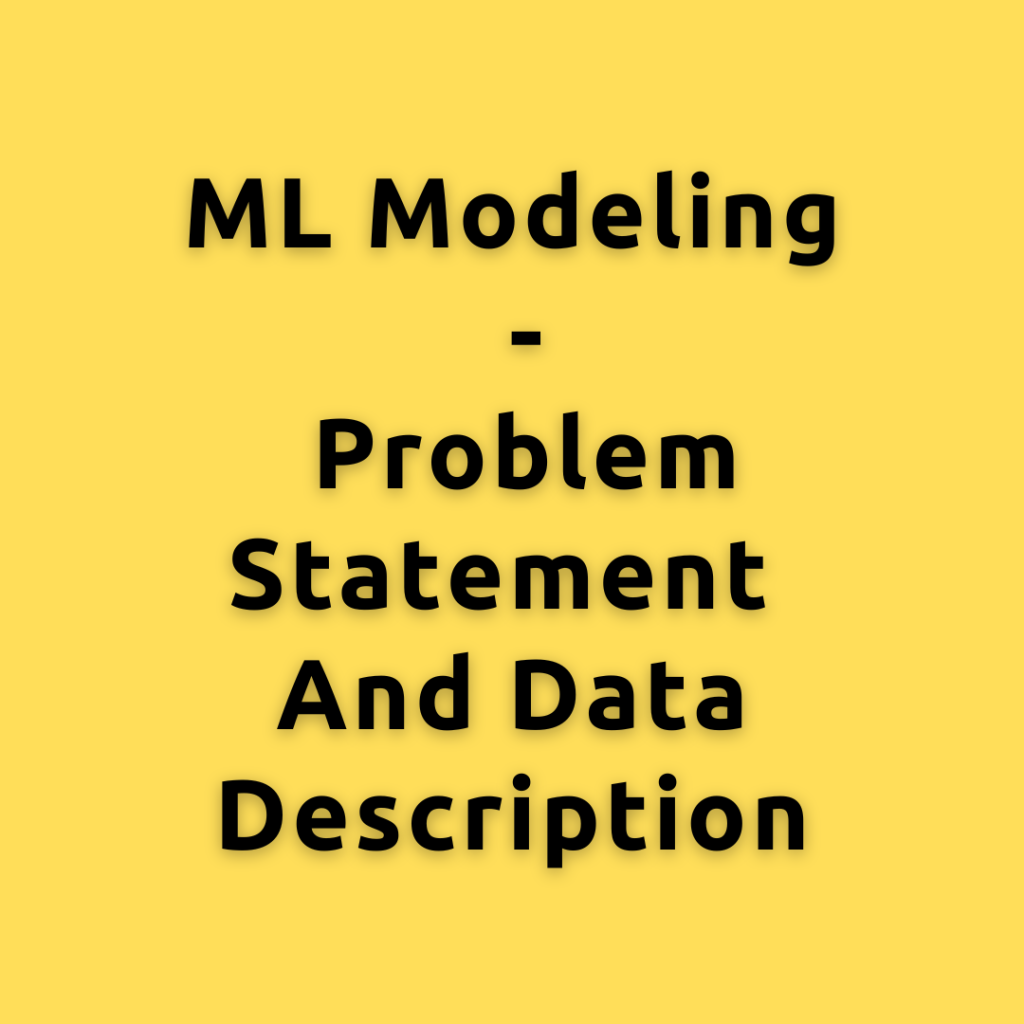## ML Modeling – Problem statement and Data description

ML modeling is the step where machine learning is used to find patterns in data and use that learned knowledge to predict an outcome. The type of ML modeling we are going to solve in this problem is called ‘Churn Modeling’. Let’s first understand the Churn modeling problem statement and then go over the data …Adaboost is one of the earliest implementations of the boosting algorithm. It forms the base of other boosting algorithms, like gradient boosting and XGBoost. This tutorial will take you through the math behind implementing this algorithm and also a practical example of using the scikit-learn Adaboost API. Contents: What is boosting? What is Adaboost? Algorithm …

## How to formulate machine learning problem

Let’s understand how to define and formulate the machine learning problem (for predictive modeling) from a business problem. This structured approach should help you apply the process to most other types of predictive modeling problems at work. Introduction Often in ML teams, you will hear from the business/company departments about the problems and issues they …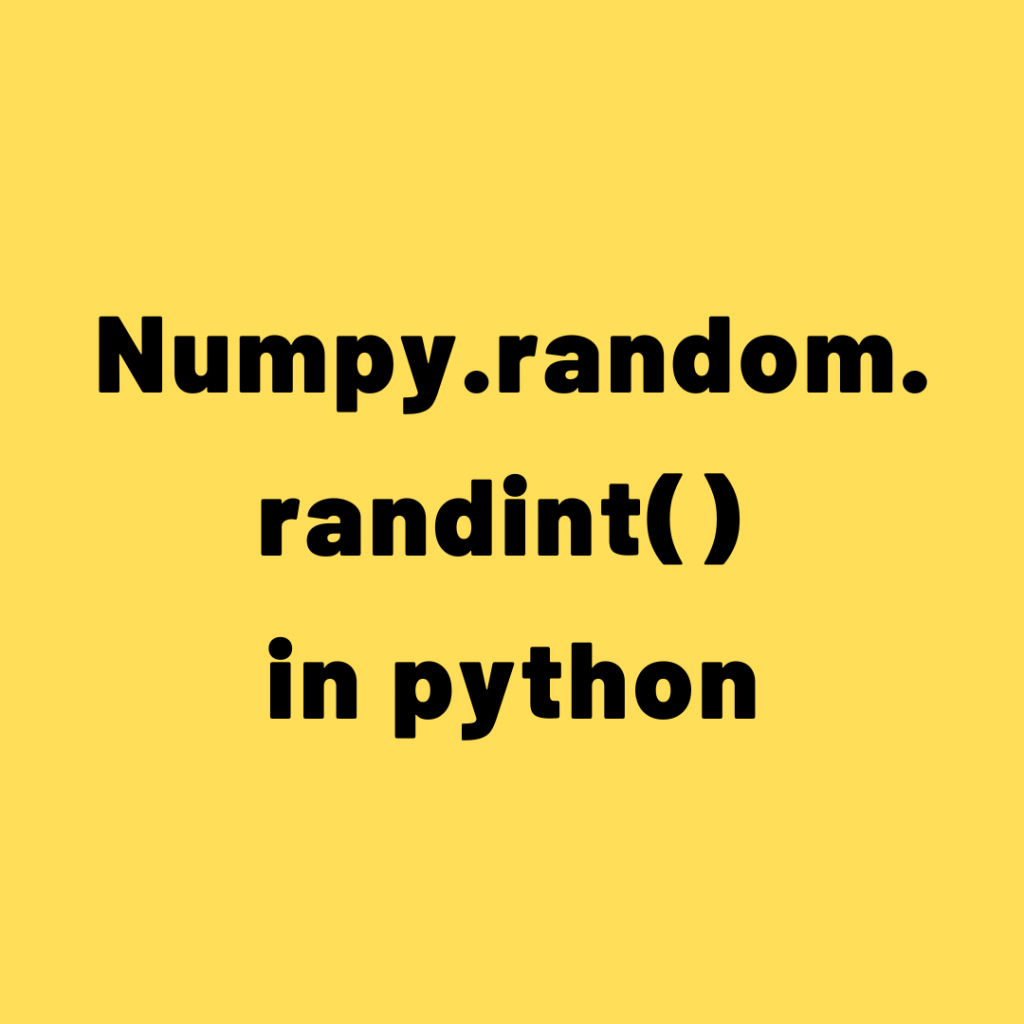## Numpy.random.randint() in python

numpy.random.randint function is used to get random integers from low to high values. The low value is included while the high value is excluded in the calculations. The output values are taken from the discrete uniform distribution of the range values. random.randint(low, high=None, size=None, dtype=int) Purpose: The numpy random randint function used for creating a …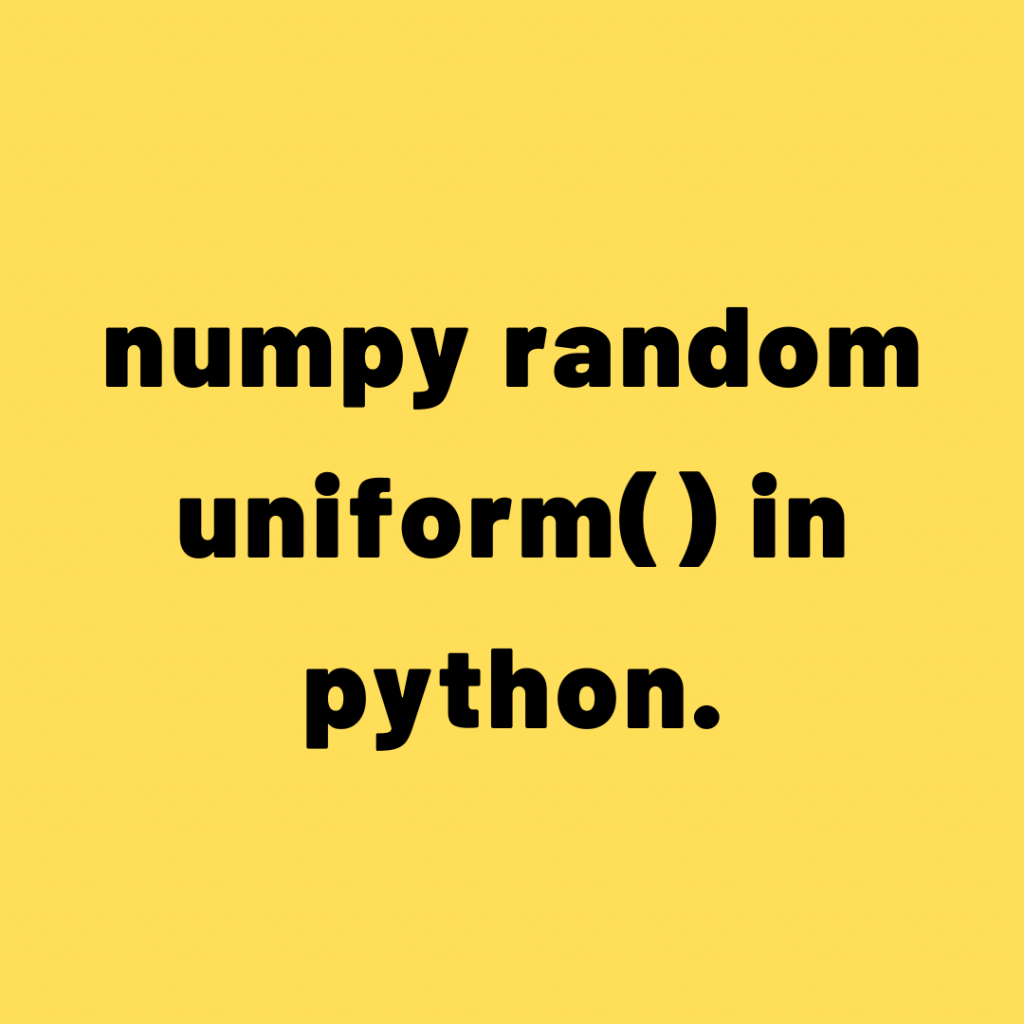## How to use numpy.random.uniform() in python.

The np.random.uniform() function is used to create an array with random samples from a uniform probability distribution of given low and high values. random.uniform(low=0.0, high=1.0, size=None) Purpose: The numpy random uniform function used for creating a numpy array with random float values from low to high interval. Parameteres: Low: float or array-like of floats,optional: Lowest …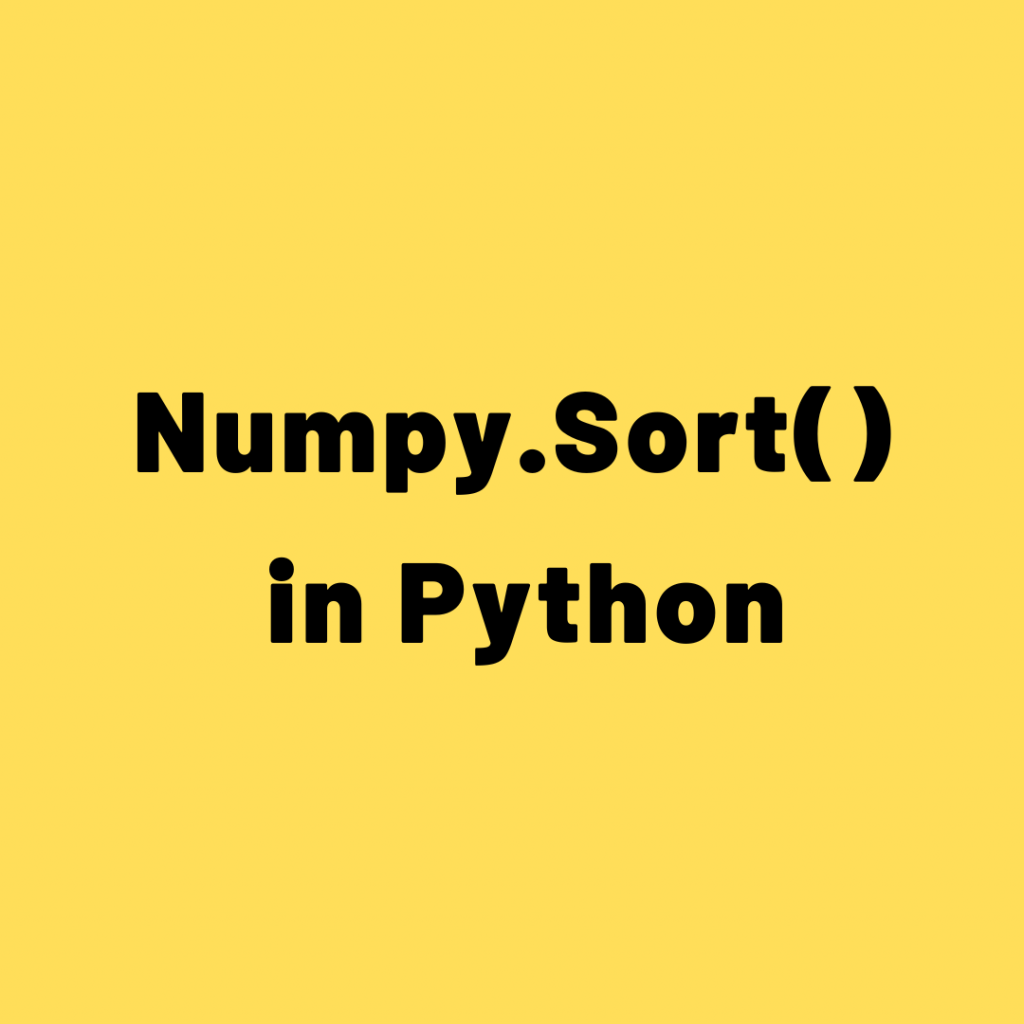## Numpy.sort() in python

The np.sort() function is used to sort the array along a specified axis. Numpy.sort (a, axis=- 1, kind=None, order=None) Purpose: This function is used for sorting the array. Parameters: arr:a:array_like array to be sorted. axis: None or int,optional Axis on which we perform the arithmetic mean if specified. otherwise, the arr will be flattened. kind: …## How to Use Numpy Where Function?

The numpy.where() function is used to filter data based on the conditions provided. These conditions can vary from being as simple as value comparisons to nested bit-wise conditions. You can also use this function to perform conditional replacements in the input data array. In this article, you will learn about the possible use cases of …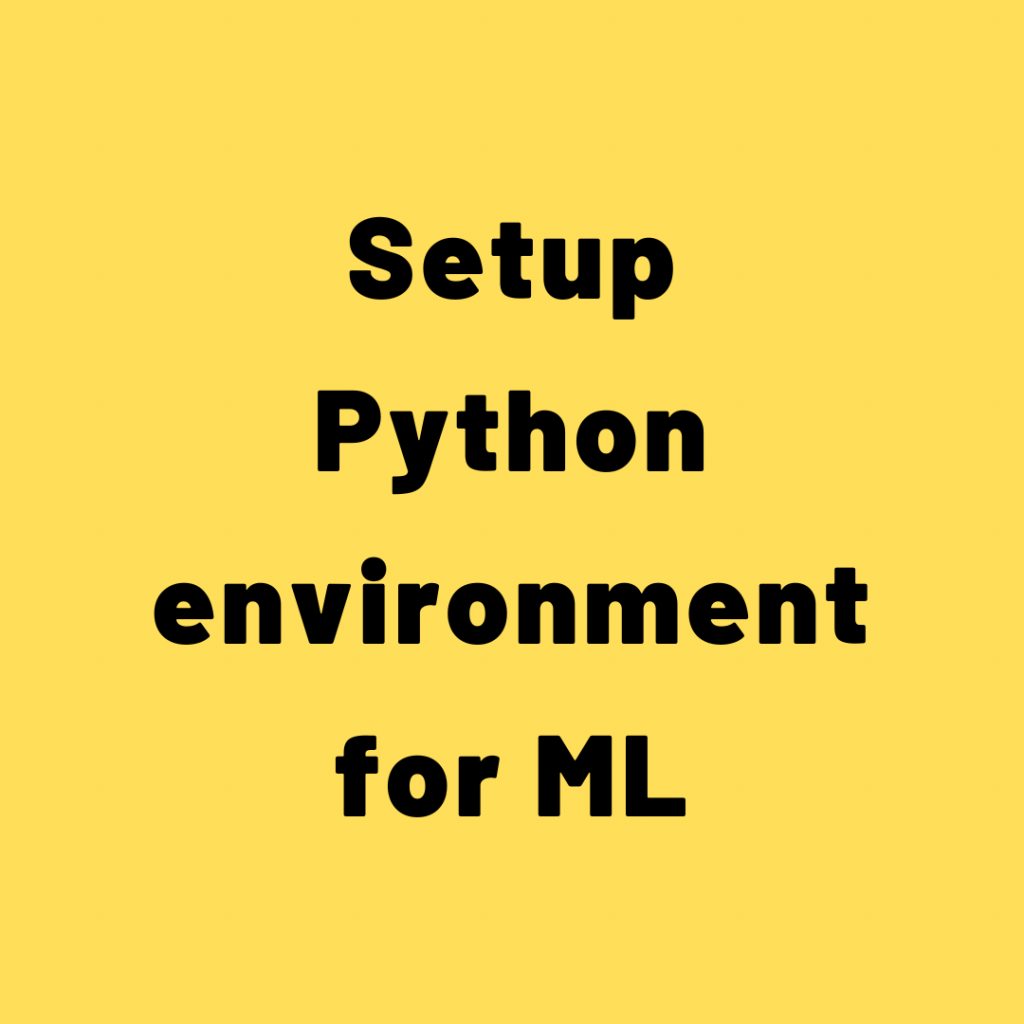## Setup Python environment for ML

Python is the most popular programming language used for AI and machine learning. Let’s see how to setup python environment for ML using anaconda. How to install Python? Simply visit Python.org, go to downloads section, download latest version that shows there and install it like you do for any other software. To do machine learning …Course Preview

## Machine Learning A-Z™: Hands-On Python & R In Data Science

### Free Sample Videos:#### Machine Learning A-Z™: Hands-On Python & R In Data Science#### Machine Learning A-Z™: Hands-On Python & R In Data Science#### Machine Learning A-Z™: Hands-On Python & R In Data Science#### Machine Learning A-Z™: Hands-On Python & R In Data Science